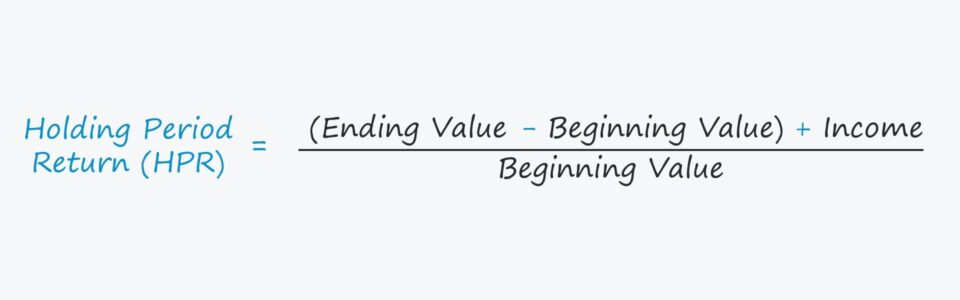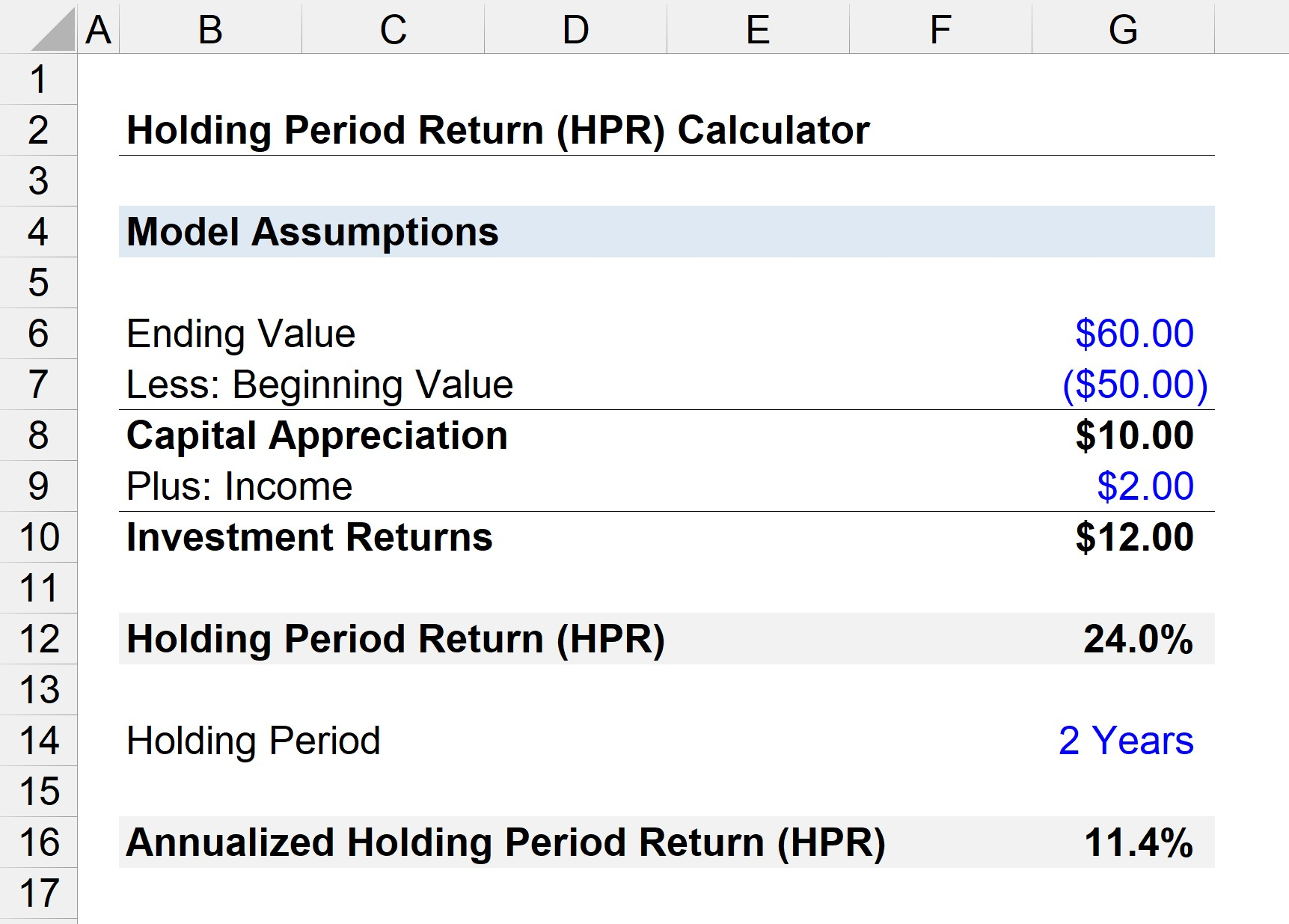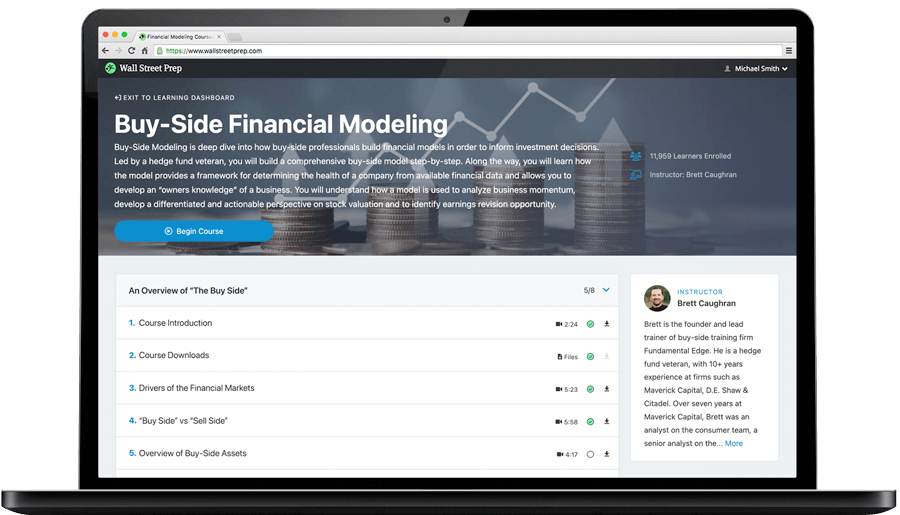Welcome to Wall Street Prep! Use code at checkout for 15% off.# Holding Period Return (HPR)

Guide to Understanding the Holding Period Return (HPR)## How to Calculate Holding Period Return (HPR)?

Conceptually, HPR refers to the return received on an investment (or portfolio of securities) throughout the period during which the investment was held.

The holding period return (HPR) metric is composed of two-income sources: capital appreciation and dividend (or interest) income, expressed as a percentage.

1. Capital Appreciation: Sale Price > Purchase Price
2. Income: Dividends and/or Interest Income

More specifically, an investor can earn returns in the form of capital appreciation (i.e. selling the investment at a price higher than the purchase price) and receive income, such as dividends or interest income.

• If the investment is in a company’s shares, dividends represent the income source of the equity shareholders.
• If the investment is in debt securities, interest would be the income received by the bondholders.

## Holding Period Return Formula (HPR)

Calculating the HPR starts by subtracting the beginning value of an investment from the ending value to arrive at the capital appreciation value, i.e. the capital gain.

The capital appreciation formula – i.e. ending value minus beginning value – measures how much an investment how grown (or declined) in price since the initial purchase.

Capital Appreciation = Ending Value – Beginning Value

A capital gain occurs if the sale price exceeds the purchase price, whereas if the security was sold for less than the initial price paid on the original date of purchase, the investment would be sold for a capital loss.

The amount of income received is then added to the capital appreciation in the next step.

The resulting figure represents the total return, i.e. the sum of the capital appreciation and income.

With the numerator calculated, the final step is to divide by the beginning investment value, as shown by the formula below.

Holding Period Return (HPR) = [(Ending Value — Beginning Value) + Income] / Beginning Value

The return can also be calculated using the following formula if the investment consists of stocks.

HPR = Capital Gains Yield + Dividend Yield

## Annualized HPR Formula

The holding period can range from a couple of days to multiple years, so annualizing the returns is necessary to compare the returns of different investments.

For instance, the absolute HPR of an investment could be less than that of another investment but be greater on an annualized basis.

Annualized HPR = (1 + Holding Period Return) ^ (1 / t) – 1

The annualized holding period return makes it easier to compare returns among investments with varying holding periods (i.e. so that they are “apples to apples”).

## Holding Period Return Calculator (HPR)

We’ll now move to a modeling exercise, which you can access by filling out the form below.Submitting...

## Step 1. Stock Capital Appreciation Calculation

Suppose you purchased one share in a public company for \$50 and held onto the investment for two years.

During the two-year holding period, the share price rose to \$60, reflecting a capital appreciation of \$10 (a 20% increase).

• Capital Appreciation = \$60 – \$50 = \$10

## Step 2. Income Earned Calculation (Shareholder Dividend)

With the first component of returns calculated – i.e. the \$10 capital appreciation – the next step is to add the total dividend income received, which we’ll assume was \$2 in total received since the date of purchase.

• \$10 + \$2 = \$12

## Step 3. Holding Period Return Calculation Analysis

The remaining step is to divide the total return by the beginning value, i.e. the \$50 purchase price.

• Holding Period Return (HPR) = \$12 / \$50 = 24%

The holding period return (HPR) on the investment is 24%, which will now be annualized using the holding period of two years.

• Annualized Holding Period Return (HPR) = (1 + 24%) ^ (1 / 2) – 1 = 11.4%Step-by-Step Online Course

### Learn Buy-Side (Hedge Fund) Modeling

Led by a former hedge fund PM (Maverick, Citadel, DE Shaw, Schonfeld), this program begins where financial modeling training ends — with a deep-dive into how buy-side analysts build financial models to make key investment decisions.